Anúncio

# Eng.Materials-mechanical-properties-6.pdf

22 de Mar de 2023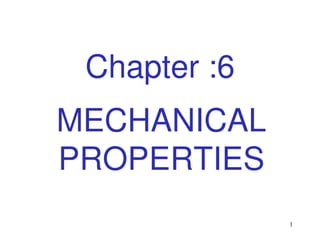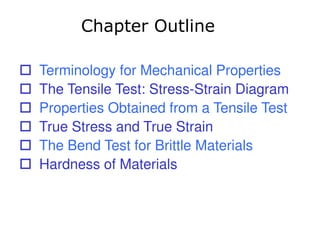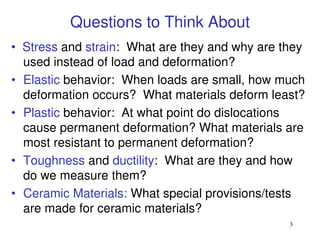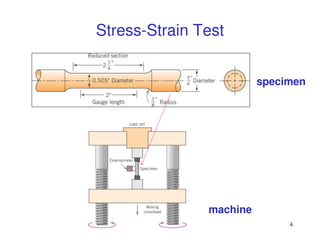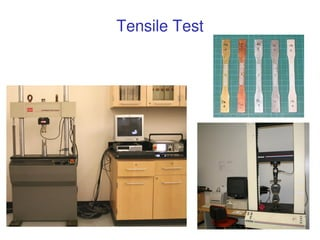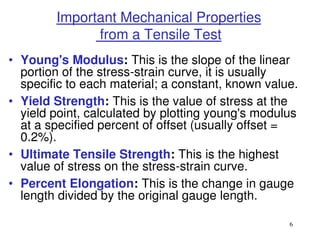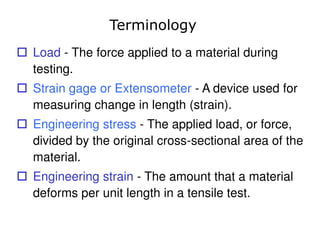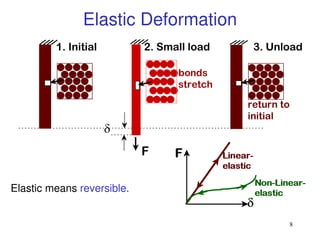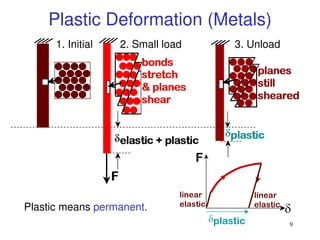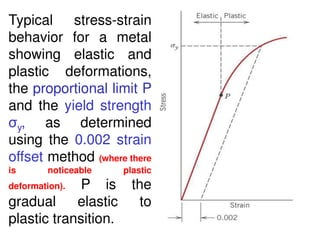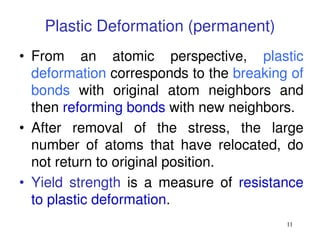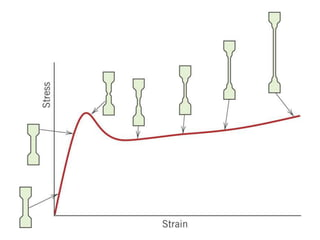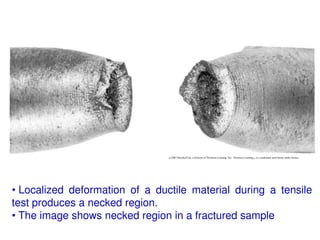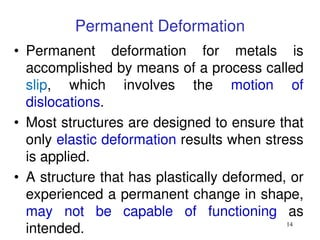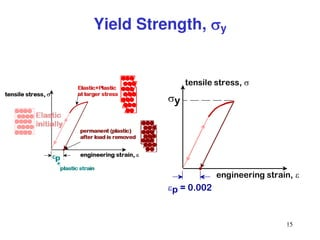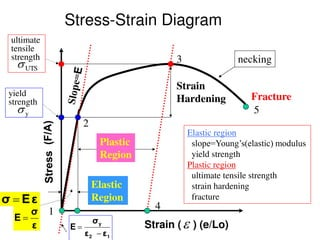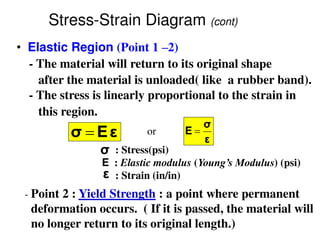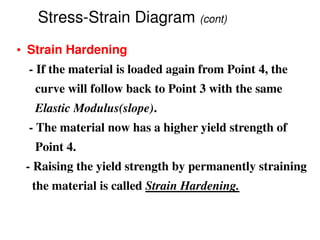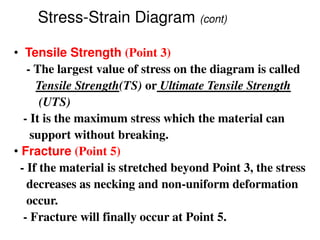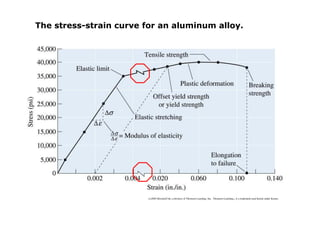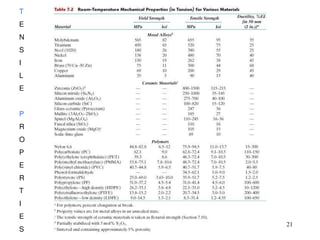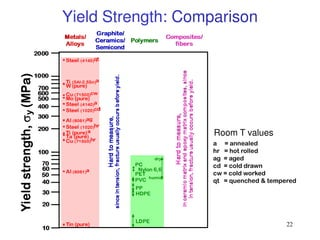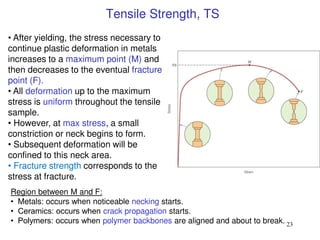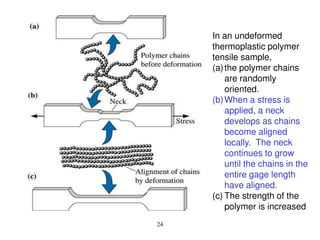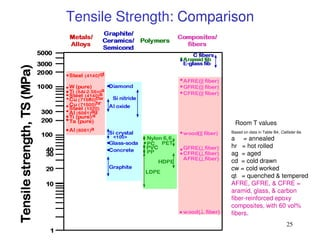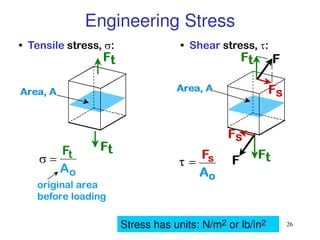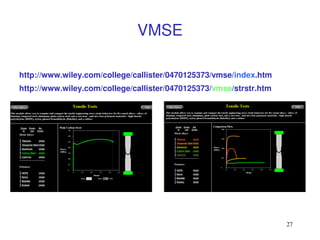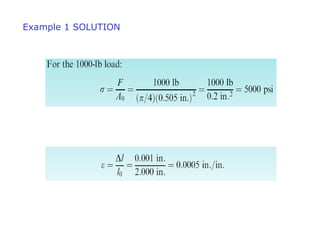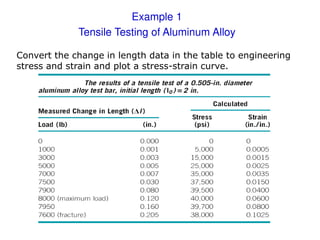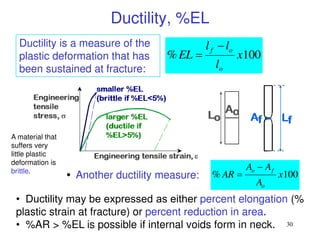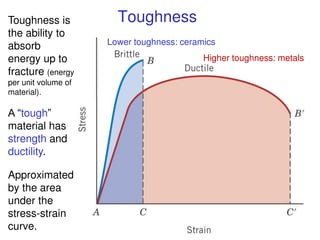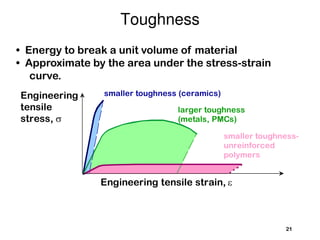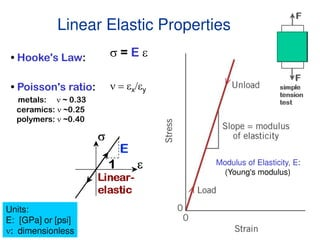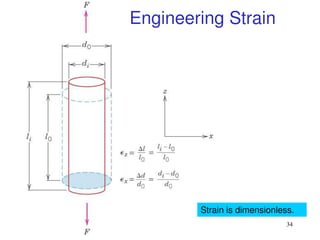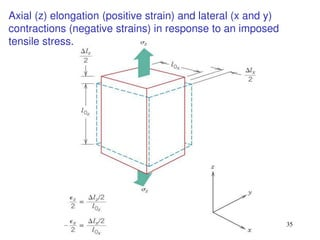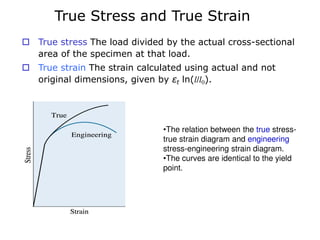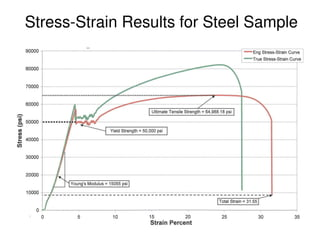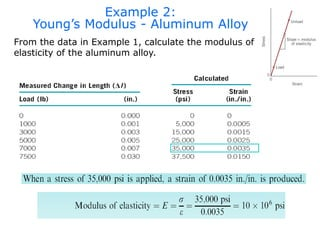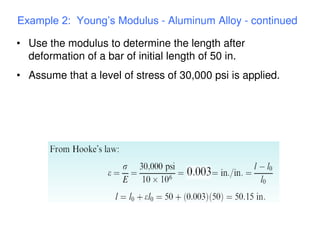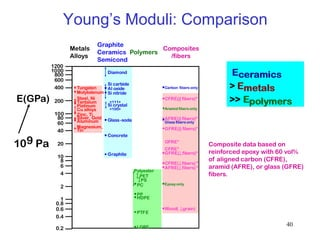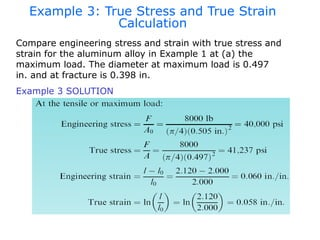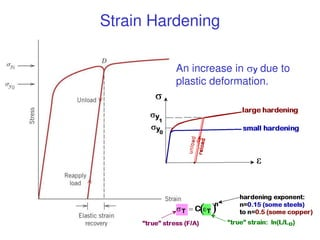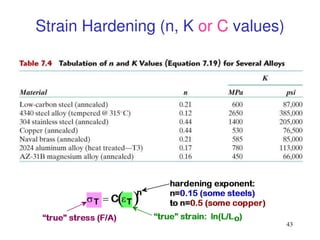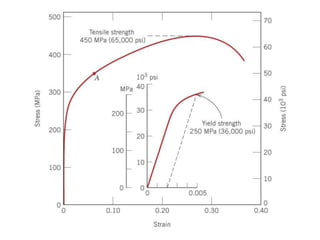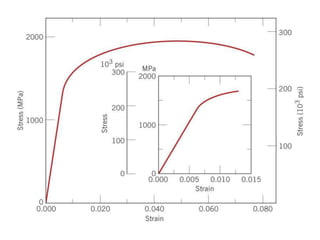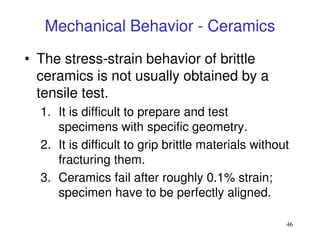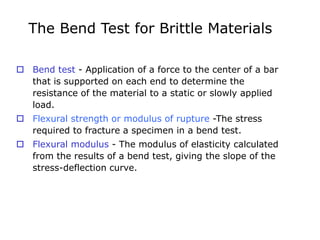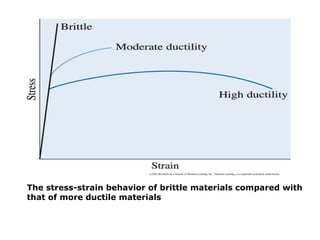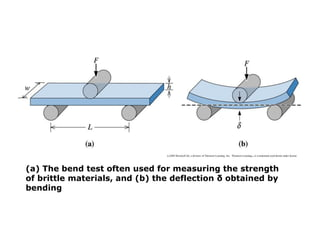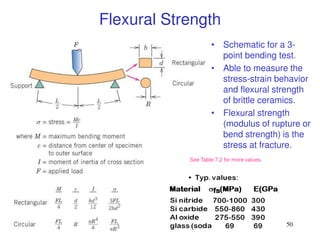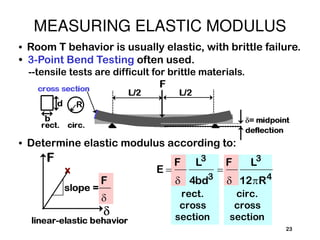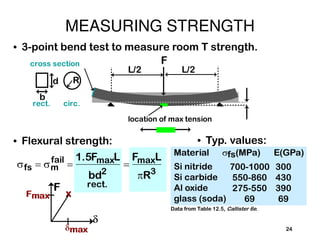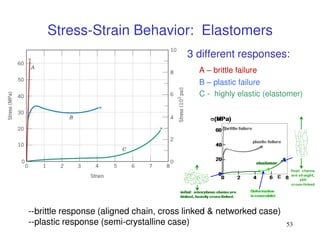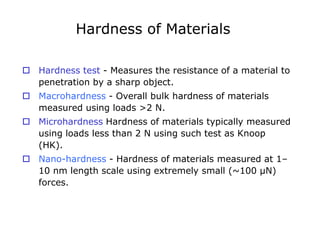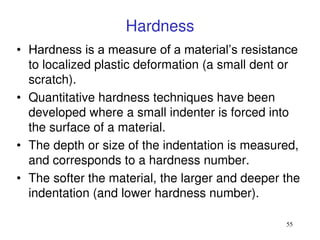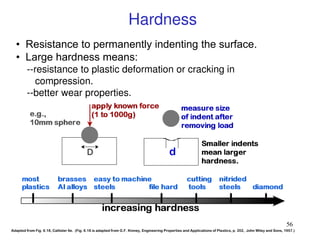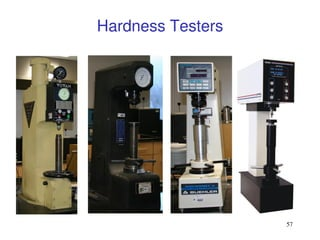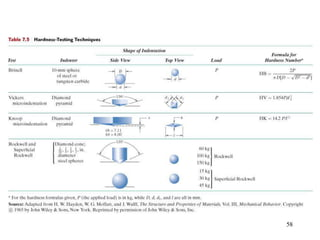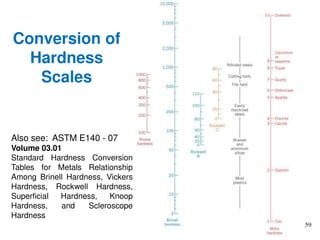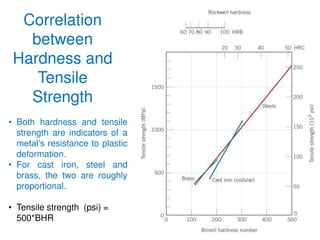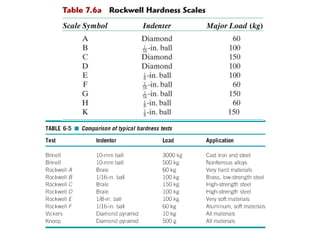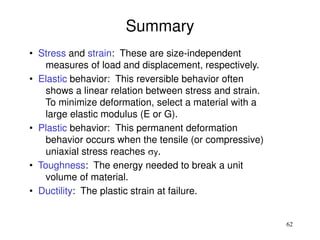1 de 62
Anúncio

### Eng.Materials-mechanical-properties-6.pdf

1. 1 Chapter :6 MECHANICAL PROPERTIES
2. Chapter Outline  Terminology for Mechanical Properties  The Tensile Test: Stress-Strain Diagram  Properties Obtained from a Tensile Test  True Stress and True Strain  The Bend Test for Brittle Materials  Hardness of Materials
3. 3 Questions to Think About • Stress and strain: What are they and why are they used instead of load and deformation? • Elastic behavior: When loads are small, how much deformation occurs? What materials deform least? • Plastic behavior: At what point do dislocations cause permanent deformation? What materials are most resistant to permanent deformation? • Toughness and ductility: What are they and how do we measure them? • Ceramic Materials: What special provisions/tests are made for ceramic materials?
4. 4 Stress-Strain Test specimen machine
5. 5 Tensile Test
6. 6 Important Mechanical Properties from a Tensile Test • Young's Modulus: This is the slope of the linear portion of the stress-strain curve, it is usually specific to each material; a constant, known value. • Yield Strength: This is the value of stress at the yield point, calculated by plotting young's modulus at a specified percent of offset (usually offset = 0.2%). • Ultimate Tensile Strength: This is the highest value of stress on the stress-strain curve. • Percent Elongation: This is the change in gauge length divided by the original gauge length.
7. Terminology  Load - The force applied to a material during testing.  Strain gage or Extensometer - A device used for measuring change in length (strain).  Engineering stress - The applied load, or force, divided by the original cross-sectional area of the material.  Engineering strain - The amount that a material deforms per unit length in a tensile test.
9. 9 1. Initial 2. Small load 3. Unload Plastic means permanent. F  linear elastic linear elastic plastic Plastic Deformation (Metals)
10. 10 Typical stress-strain behavior for a metal showing elastic and plastic deformations, the proportional limit P and the yield strength σy, as determined using the 0.002 strain offset method (where there is noticeable plastic deformation). P is the gradual elastic to plastic transition.
11. 11 Plastic Deformation (permanent) • From an atomic perspective, plastic deformation corresponds to the breaking of bonds with original atom neighbors and then reforming bonds with new neighbors. • After removal of the stress, the large number of atoms that have relocated, do not return to original position. • Yield strength is a measure of resistance to plastic deformation.
12. 12
13. (c)2003 Brooks/Cole, a division of Thomson Learning, Inc. Thomson Learning™ is a trademark used herein under license. • Localized deformation of a ductile material during a tensile test produces a necked region. • The image shows necked region in a fractured sample
14. 14 Permanent Deformation • Permanent deformation for metals is accomplished by means of a process called slip, which involves the motion of dislocations. • Most structures are designed to ensure that only elastic deformation results when stress is applied. • A structure that has plastically deformed, or experienced a permanent change in shape, may not be capable of functioning as intended.
15. 15 tensile stress,  engineering strain,  y p = 0.002 Yield Strength, y
16. Stress-Strain Diagram Strain ( ) (e/Lo) 4 1 2 3 5 Elastic Region Plastic Region Strain Hardening Fracture ultimate tensile strength Elastic region slope=Young’s(elastic) modulus yield strength Plastic region ultimate tensile strength strain hardening fracture necking yield strength UTS  y  ε E σ  ε σ E   1 2 y ε ε σ E  
17. Stress-Strain Diagram (cont) • Elastic Region (Point 1 –2) - The material will return to its original shape after the material is unloaded( like a rubber band). - The stress is linearly proportional to the strain in this region. ε E σ  : Stress(psi) E : Elastic modulus (Young’s Modulus) (psi) : Strain (in/in) σ ε - Point 2 : Yield Strength : a point where permanent deformation occurs. ( If it is passed, the material will no longer return to its original length.) ε σ E  or
18. • Strain Hardening - If the material is loaded again from Point 4, the curve will follow back to Point 3 with the same Elastic Modulus(slope). - The material now has a higher yield strength of Point 4. - Raising the yield strength by permanently straining the material is called Strain Hardening. Stress-Strain Diagram (cont)
19. • Tensile Strength (Point 3) - The largest value of stress on the diagram is called Tensile Strength(TS) or Ultimate Tensile Strength (UTS) - It is the maximum stress which the material can support without breaking. • Fracture (Point 5) - If the material is stretched beyond Point 3, the stress decreases as necking and non-uniform deformation occur. - Fracture will finally occur at Point 5. Stress-Strain Diagram (cont)
20. (c)2003 Brooks/Cole, a division of Thomson Learning, Inc. Thomson Learning™ is a trademark used herein under license. The stress-strain curve for an aluminum alloy.
21. 21 T E N S I L E P R O P E R T I E S
22. 22 Room T values a = annealed hr = hot rolled ag = aged cd = cold drawn cw = cold worked qt = quenched & tempered Yield Strength: Comparison
23. 23 • After yielding, the stress necessary to continue plastic deformation in metals increases to a maximum point (M) and then decreases to the eventual fracture point (F). • All deformation up to the maximum stress is uniform throughout the tensile sample. • However, at max stress, a small constriction or neck begins to form. • Subsequent deformation will be confined to this neck area. • Fracture strength corresponds to the stress at fracture. Region between M and F: • Metals: occurs when noticeable necking starts. • Ceramics: occurs when crack propagation starts. • Polymers: occurs when polymer backbones are aligned and about to break. Tensile Strength, TS
24. 24 In an undeformed thermoplastic polymer tensile sample, (a)the polymer chains are randomly oriented. (b)When a stress is applied, a neck develops as chains become aligned locally. The neck continues to grow until the chains in the entire gage length have aligned. (c) The strength of the polymer is increased
25. 25 Room T values Based on data in Table B4, Callister 6e. a = annealed hr = hot rolled ag = aged cd = cold drawn cw = cold worked qt = quenched & tempered AFRE, GFRE, & CFRE = aramid, glass, & carbon fiber-reinforced epoxy composites, with 60 vol% fibers. Tensile Strength: Comparison
26. 26 • Tensile stress, : • Shear stress, t:   Ft Ao original area before loading Stress has units: N/m2 or lb/in2 Engineering Stress
27. 27 VMSE http://www.wiley.com/college/callister/0470125373/vmse/strstr.htm http://www.wiley.com/college/callister/0470125373/vmse/index.htm
28. Example 1 SOLUTION
29. Example 1 Tensile Testing of Aluminum Alloy Convert the change in length data in the table to engineering stress and strain and plot a stress-strain curve.
30. 30 • Another ductility measure: 100 % x A A A AR o f o   • Ductility may be expressed as either percent elongation (% plastic strain at fracture) or percent reduction in area. • %AR > %EL is possible if internal voids form in neck. 100 % x l l l EL o o f   Ductility, %EL Ductility is a measure of the plastic deformation that has been sustained at fracture: A material that suffers very little plastic deformation is brittle.
31. 31 Toughness Lower toughness: ceramics Higher toughness: metals Toughness is the ability to absorb energy up to fracture (energy per unit volume of material). A “tough” material has strength and ductility. Approximated by the area under the stress-strain curve.
32. • Energy to break a unit volume of material • Approximate by the area under the stress-strain curve. 21 smaller toughness- unreinforced polymers Engineering tensile strain,  Engineering tensile stress,  smaller toughness (ceramics) larger toughness (metals, PMCs) Toughness
33. 33 Linear Elastic Properties Modulus of Elasticity, E: (Young's modulus) • Hooke's Law:  = E  • Poisson's ratio: metals: n ~ 0.33 ceramics: n ~0.25 polymers: n ~0.40 Units: E: [GPa] or [psi] n: dimensionless n  x/y
34. 34 Engineering Strain Strain is dimensionless.
35. 35 Axial (z) elongation (positive strain) and lateral (x and y) contractions (negative strains) in response to an imposed tensile stress.
36. True Stress and True Strain  True stress The load divided by the actual cross-sectional area of the specimen at that load.  True strain The strain calculated using actual and not original dimensions, given by εt ln(l/l0). •The relation between the true stress- true strain diagram and engineering stress-engineering strain diagram. •The curves are identical to the yield point.
37. 37 Stress-Strain Results for Steel Sample
38. Example 2: Young’s Modulus - Aluminum Alloy From the data in Example 1, calculate the modulus of elasticity of the aluminum alloy.
39. • Use the modulus to determine the length after deformation of a bar of initial length of 50 in. • Assume that a level of stress of 30,000 psi is applied. Example 2: Young’s Modulus - Aluminum Alloy - continued
40. 40 0.2 8 0.6 1 Magnesium, Aluminum Platinum Silver, Gold Tantalum Zinc, Ti Steel, Ni Molybdenum Graphite Si crystal Glass-soda Concrete Si nitride Al oxide PC Wood( grain) AFRE( fibers)* CFRE* GFRE* Glass fibers only Carbon fibers only Aramid fibers only Epoxy only 0.4 0.8 2 4 6 10 20 40 60 80 100 200 600 800 1000 1200 400 Tin Cu alloys Tungsten <100> <111> Si carbide Diamond PTFE HDPE LDPE PP Polyester PS PET CFRE( fibers)* GFRE( fibers)* GFRE(|| fibers)* AFRE(|| fibers)* CFRE(|| fibers)* Metals Alloys Graphite Ceramics Semicond Polymers Composites /fibers E(GPa) 109 Pa Composite data based on reinforced epoxy with 60 vol% of aligned carbon (CFRE), aramid (AFRE), or glass (GFRE) fibers. Young’s Moduli: Comparison
41. Example 3: True Stress and True Strain Calculation Compare engineering stress and strain with true stress and strain for the aluminum alloy in Example 1 at (a) the maximum load. The diameter at maximum load is 0.497 in. and at fracture is 0.398 in. Example 3 SOLUTION
42. Strain Hardening An increase in y due to plastic deformation.
43. 43 Strain Hardening (n, K or C values)
44. 46 Mechanical Behavior - Ceramics • The stress-strain behavior of brittle ceramics is not usually obtained by a tensile test. 1. It is difficult to prepare and test specimens with specific geometry. 2. It is difficult to grip brittle materials without fracturing them. 3. Ceramics fail after roughly 0.1% strain; specimen have to be perfectly aligned.
45. The Bend Test for Brittle Materials  Bend test - Application of a force to the center of a bar that is supported on each end to determine the resistance of the material to a static or slowly applied load.  Flexural strength or modulus of rupture -The stress required to fracture a specimen in a bend test.  Flexural modulus - The modulus of elasticity calculated from the results of a bend test, giving the slope of the stress-deflection curve.
46. (c)2003 Brooks/Cole, a division of Thomson Learning, Inc. Thomson Learning™ is a trademark used herein under license. The stress-strain behavior of brittle materials compared with that of more ductile materials
47. (c)2003 Brooks/Cole, a division of Thomson Learning, Inc. Thomson Learning™ is a trademark used herein under license. (a) The bend test often used for measuring the strength of brittle materials, and (b) the deflection δ obtained by bending
48. 50 • Schematic for a 3- point bending test. • Able to measure the stress-strain behavior and flexural strength of brittle ceramics. • Flexural strength (modulus of rupture or bend strength) is the stress at fracture. Flexural Strength See Table 7.2 for more values.
49. 23 • Room T behavior is usually elastic, with brittle failure. • 3-Point Bend Testing often used. --tensile tests are difficult for brittle materials. • Determine elastic modulus according to: E  F  L3 4bd3  F  L3 12R4 rect. cross section circ. cross section MEASURING ELASTIC MODULUS
50. 24 • 3-point bend test to measure room T strength. F L/2 L/2 cross section R b d rect. circ. location of max tension • Flexural strength: rect. fs  m fail  1.5FmaxL bd2  FmaxL R3 • Typ. values: Material fs(MPa) E(GPa) Si nitride Si carbide Al oxide glass (soda) 700-1000 550-860 275-550 69 300 430 390 69 Data from Table 12.5, Callister 6e. MEASURING STRENGTH
51. 53 --brittle response (aligned chain, cross linked & networked case) --plastic response (semi-crystalline case) Stress-Strain Behavior: Elastomers 3 different responses: A – brittle failure B – plastic failure C - highly elastic (elastomer)
52. Hardness of Materials  Hardness test - Measures the resistance of a material to penetration by a sharp object.  Macrohardness - Overall bulk hardness of materials measured using loads >2 N.  Microhardness Hardness of materials typically measured using loads less than 2 N using such test as Knoop (HK).  Nano-hardness - Hardness of materials measured at 1– 10 nm length scale using extremely small (~100 µN) forces.
53. 55 Hardness • Hardness is a measure of a material’s resistance to localized plastic deformation (a small dent or scratch). • Quantitative hardness techniques have been developed where a small indenter is forced into the surface of a material. • The depth or size of the indentation is measured, and corresponds to a hardness number. • The softer the material, the larger and deeper the indentation (and lower hardness number).
54. 56 • Resistance to permanently indenting the surface. • Large hardness means: --resistance to plastic deformation or cracking in compression. --better wear properties. Adapted from Fig. 6.18, Callister 6e. (Fig. 6.18 is adapted from G.F. Kinney, Engineering Properties and Applications of Plastics, p. 202, John Wiley and Sons, 1957.) Hardness
55. 57 Hardness Testers
56. 58
57. 59 Conversion of Hardness Scales Also see: ASTM E140 - 07 Volume 03.01 Standard Hardness Conversion Tables for Metals Relationship Among Brinell Hardness, Vickers Hardness, Rockwell Hardness, Superficial Hardness, Knoop Hardness, and Scleroscope Hardness
58. 60 Correlation between Hardness and Tensile Strength • Both hardness and tensile strength are indicators of a metal’s resistance to plastic deformation. • For cast iron, steel and brass, the two are roughly proportional. • Tensile strength (psi) = 500*BHR
59. 62 • Stress and strain: These are size-independent measures of load and displacement, respectively. • Elastic behavior: This reversible behavior often shows a linear relation between stress and strain. To minimize deformation, select a material with a large elastic modulus (E or G). • Plastic behavior: This permanent deformation behavior occurs when the tensile (or compressive) uniaxial stress reaches y. • Toughness: The energy needed to break a unit volume of material. • Ductility: The plastic strain at failure. Summary
Anúncio